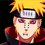# Coulomb's LawCoulomb's law is a law of physics describing the electrostatic interaction between electrically charged particles. Was formulated and first published in $1783$ by French physicist Charles Augustin de Coulomb and was essential to the development of the study of electricity. This law states that the magnitude of the force between two punctate electrical charges ($q_1$ and $q_2$) is directly proportional to the product of the absolute values ​​(modules) of the two charges and inversely proportional to the square of the distance r between them. This force can be attractive or repulsive depending on the sign of the charges. It is attractive if the charges have opposite signs. It is repulsive if the charges have the same sign.Diagram depicting the basic mechanism of Coulomb's law. The like charges repel and opposite charges attract Once detailed measurements using a torsion balance, concluded that the Coulomb force is fully described by the following equation:Where :Is the Force In Newtons $(N)$$C^2 N^{-1} M{-2}$ Is the Permittivity of free space

$r$ Is the Distance between the Two Point charges in Meters $(m)$ and $Q_1$ and $Q_2$, respective load values ​​in Coulombs $(C)$ .Is the Vector that Indicates the Direction in Which the Electric Force points.

Sometimes it replaces the factor $\frac{1}{4 \pi \epsilon_o }$ by $k$ , Coulomb's Constant, with $k$The previous notation is a compact vector notation, which is not specified any coordinate system. If the load $1$ is the source and the load at point $2$ with Cartesian coordinates $(x, y, z)$ the Coulomb force takes the form:How to charge a Coulomb (C 1) is very large, it is customary to use submultiples of this unit. Thus we have:

$1$ Milicoulomb = $10^{-3} C$

$1$ Microcoulomb = $10^{-6} C$

$1$ Nanocoulomb = $10^{-9} C$

$1$ Picocoulomb = $10^{-12} C$

Remembering that $C= 6,25 \times 10^{18}$ Electrons

# Examples

Problem I

Two particles of electric charges

$Q = 4.0 × 10^ {-16} C$ and $q = 6.0 × 10^{-16} C$

vacuum are separated by a distance of $3,0 \times 10^{-9}m$.

Where $k = 9.0 \times 10^9$ , the intensity of the force of interaction between them, in newtons, Is :

$a) 1,2 \times 10{-5}$

$b) 1,8 \times 10{-4}$

$c) 2,0 \times 10^{-4}$

$d) 24 \times 10^{-5}$

$e) 30 \times 10^{-4}$

Solution :

$F = \frac{k \times Q_1 \times Q_2}{d^2}$

$F = \frac{9,0 \times 10^9 \times 4,0 \times 10^{-16} \times 6,0 \times 10^{ -16}}{(3,0 \times 10^{-9})^2}$

$F = \frac{9,0 \times 10^9 \times 4,0 \times 10^{-16} \times 6,0 \times 10^{-16}}{(3,0 \times 10^{-9} \times 3,0 \times 10^{-9})}$

$F = \frac{3,0 \times 10^9 \times 4,0 \times 10^){-16} \times 2,0 \times 10^{-16}}{10^{-18} }$

$F = \frac{24 \times 10^9 \times 10^{-16} \times 10^{-16}}{10^{-18} }$

$F = \frac{24 \times 10^{-23}}{10^{-18} }$

$F = \boxed{24 \times 10^{-5} N}$

You Can Watch these Videos for See More Examples:

Coulomb's Law I

Coulomb's Law II

Coulomb's Law III

Coulomb's Law IVNote by Gabriel Merces
6 years, 4 months ago

This discussion board is a place to discuss our Daily Challenges and the math and science related to those challenges. Explanations are more than just a solution — they should explain the steps and thinking strategies that you used to obtain the solution. Comments should further the discussion of math and science.

When posting on Brilliant:

• Use the emojis to react to an explanation, whether you're congratulating a job well done , or just really confused .
• Ask specific questions about the challenge or the steps in somebody's explanation. Well-posed questions can add a lot to the discussion, but posting "I don't understand!" doesn't help anyone.
• Try to contribute something new to the discussion, whether it is an extension, generalization or other idea related to the challenge.

MarkdownAppears as
*italics* or _italics_ italics
**bold** or __bold__ bold
- bulleted- list
• bulleted
• list
1. numbered2. list
1. numbered
2. list
Note: you must add a full line of space before and after lists for them to show up correctly
paragraph 1paragraph 2

paragraph 1

paragraph 2

[example link](https://brilliant.org)example link
> This is a quote
This is a quote
    # I indented these lines
# 4 spaces, and now they show
# up as a code block.

print "hello world"
# I indented these lines
# 4 spaces, and now they show
# up as a code block.

print "hello world"
MathAppears as
Remember to wrap math in $$ ... $$ or $ ... $ to ensure proper formatting.
2 \times 3 $2 \times 3$
2^{34} $2^{34}$
a_{i-1} $a_{i-1}$
\frac{2}{3} $\frac{2}{3}$
\sqrt{2} $\sqrt{2}$
\sum_{i=1}^3 $\sum_{i=1}^3$
\sin \theta $\sin \theta$
\boxed{123} $\boxed{123}$

Sort by:

Really well explained

- 6 years, 4 months ago

Thanks ! This Is Very Important for Me !

- 6 years, 4 months ago

Hello, try visiting http://sciencefront.webs.com for some cool science stuff for kids...

- 6 years, 3 months ago

very interesting

- 6 years, 3 months ago

interesting

- 6 years, 3 months ago

@Gabriel Merces Fix k in the example. It is not dimensionless.

$k=9.0\times{}10^{9} \frac{\text{ N m}^2}{\text{C}^2}$

- 6 years, 2 months ago

I wonder why they define $C=6.25\times10^{18}$ electrons. I mean for example there is a reason for $1\text{ mol}=6.02\times10^{23}$, but what about Coulomb? I hope I'm not asking silly questions...

- 6 years, 4 months ago

• The Coulomb Is the Unit of Electric Charge by the International System (SI).

• $1$ Coulomb is the Amount of Electric Charge carried by the Current of $1$ ampere per $1$ second.

- 6 years, 4 months ago

So I've just misunderstood the definition of Coulomb, Thanks!

- 6 years, 4 months ago

By the way, great note!

- 6 years, 4 months ago

@Christopher Boo Sorry this is off topic but how did you find brilliant squared? Satisfactory?

- 6 years, 4 months ago

Well, at first I thought the Practice Maps will be great, then it turns out well too! And the save sets feature is awesome! :)

- 6 years, 4 months ago

i can't understand the coulomb force with cartesian coordinates

- 6 years, 4 months ago